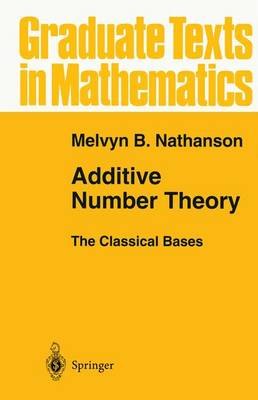•Additive Number Theory The Classical Bases - Graduate Texts in Mathematics 164 (Paperback)

(author)
£69.99
Paperback 342 Pages / Published: 19/11/2010
• We can order this

Usually dispatched within 3 weeks

[Hilbert's] style has not the terseness of many of our modem authors in mathematics, which is based on the assumption that printer's labor and paper are costly but the reader's effort and time are not. H. Weyl  The purpose of this book is to describe the classical problems in additive number theory and to introduce the circle method and the sieve method, which are the basic analytical and combinatorial tools used to attack these problems. This book is intended for students who want to lel?Ill additive number theory, not for experts who already know it. For this reason, proofs include many "unnecessary" and "obvious" steps; this is by design. The archetypical theorem in additive number theory is due to Lagrange: Every nonnegative integer is the sum of four squares. In general, the set A of nonnegative integers is called an additive basis of order h if every nonnegative integer can be written as the sum of h not necessarily distinct elements of A. Lagrange 's theorem is the statement that the squares are a basis of order four. The set A is called a basis offinite order if A is a basis of order h for some positive integer h. Additive number theory is in large part the study of bases of finite order. The classical bases are the squares, cubes, and higher powers; the polygonal numbers; and the prime numbers. The classical questions associated with these bases are Waring's problem and the Goldbach conjecture.

Publisher: Springer-Verlag New York Inc.
ISBN: 9781441928481
Number of pages: 342
Weight: 551 g
Dimensions: 235 x 155 x 18 mm
Edition: Softcover reprint of hardcover 1st ed. 1996

MEDIA REVIEWS

From the reviews:

"This book provides a very thorough exposition of work to date on two classical problems in additive number theory ... . is aimed at students who have some background in number theory and a strong background in real analysis. A novel feature of the book, and one that makes it very easy to read, is that all the calculations are written out in full - there are no steps `left to the reader'. ... The book also includes a large number of exercises ... ." (Allen Stenger, The Mathematical Association of America, August, 2010)